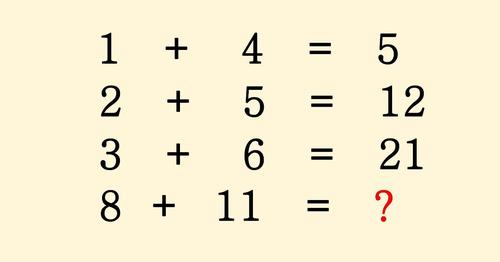# People Are Having A Really Difficult Time Solving This Math Puzzle. Can You?

If one of your responses was 40, you were entirely accurate!First problem: 1 + 4 = 5

Second problem: 2 + 5 (adding the previous sum, 5) = 12

Third problem: 3 + 9 (adding the previous sum, 12) = 21

Fourth problem: 8 + 11 (adding the previous sum, 21) = 40

But what about the other option?!

.

.

.

.

.

Are you all set?

The answer to the second question is… 96!

First problem: 1 x 4 + 1 = 5

Second problem: 2 x 5 + 2 = 12

Third problem: 3 x 6 + 3 = 21

Fourth problem: 8 x 11 + 8 = 96